Our World Statistics Day conversations have been a great reminder of how much statistics can inform our lives. Do you have an example of how statistics has made a difference in your life? Share your story with the Community!
The QbD Column: Mixture designs

Editor's note: This post is by @ronkenett, Anat Reiner-Benaim and @david_s of the KPA Group. It is part of a series of blog posts called The QbD Column. For more information on the authors, click the authors' community names above.Ron KenettDavid SteinbergAnat Reiner-Benaim

Scientists in the pharmaceutical industry must often determine product formulations. The properties of a formulation, or mixture, are usually a function of the relative proportions of the ingredients rather than their absolute amounts. So, in experiments with mixtures, a factorʹs value is its proportion in the mixture, which must fall between zero and one. In some cases tighter constraints are needed. For example if, as a minimum, 10% of component X1 is necessary to achieve a reaction, we must specify X1>0.10. In all cases, the sum of the proportions in any mixture recipe is one.

Designs for mixture components are fundamentally different from other experiments. In most experiments, the setting of one factor varies independently of any other factor. Thus, for example, the design can guarantee that factors are orthogonal to one another. With mixtures, it is impossible to vary one factor independently of all the others. When you change the proportion of one ingredient, the proportion of one or more other ingredients must also change to compensate. This simple fact has a profound effect on every aspect of experimentation with mixtures: the factor space, the design properties, and the interpretation of the results.

JMP provides several tools for designing mixture experiments. The DOE platform includes a mixture designer that supports experiments in which all the factors are components in a mixture. It includes several classical mixture design approaches, such as simplex, extreme vertices, and lattice. For the extreme vertices approach it also incorporates linear inequality constraints that permit limiting the geometry of the mixture factor space to feasible experimental regions. Many mixture experiments also include process variables, such as the temperature or the mixing time. The JMP Custom Design tool is ideal for these more complex settings.

### What is a ternary plot?

Mixture designs have an interesting geometry. With three factors, the factor level combinations are presented in a ternary plot, which is a two-dimensional representation of three components. Figure 1 presents a ternary plot for three mixture combinations, each made from three components. The mixtures represented are:

• [0.1, 0.1, 0.8]
• [0.1, 0.8, 0.1]
• [0.8, 0.1, 0.1]

To determine the component values on the plot, lines parallel to each edge of the triangle lead to scales giving the values of the components representing the perpendicular projections. For example, the concentrations of the components for point 1 are 0.1 for X1, 0.1 for X2 and 0.8 for X3 (these values are marked as circles on Figure 1).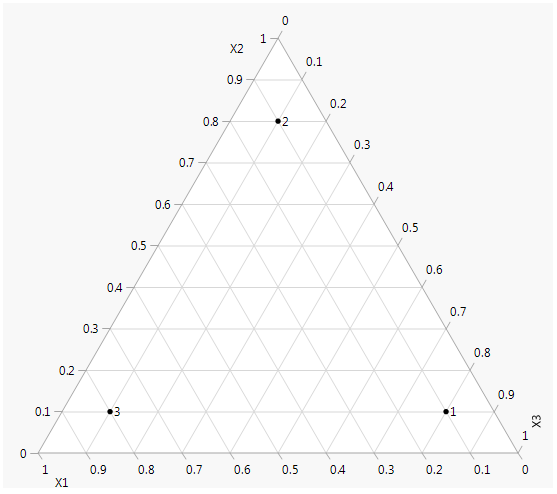Figure 1: Ternary plot of mixtures with three components

With more than three components, the feasible region for the factor settings in a mixture design takes the form of a simplex. There are a number of ways to set up ternary plots to show the mixtures used in the design. The default in JMP is to show plots for each two-component projection; the third axis in each plot shows the fraction of the mixture devoted to all the other components. Figure 2 shows such a set of plots for an experiment with 4 components. There is one plot for each of the six two-factor projections. The lower-right vertex is always labeled “Others” and corresponds to the combined amount of the other two components.

• [0.1, 0.1, 0.1, 0.7]
• [0.1, 0.7, 0.1, 0.1]
• [0.1, 0.1, 0.7, 0.1]
• [0.7, 0.1, 0.1, 0.1]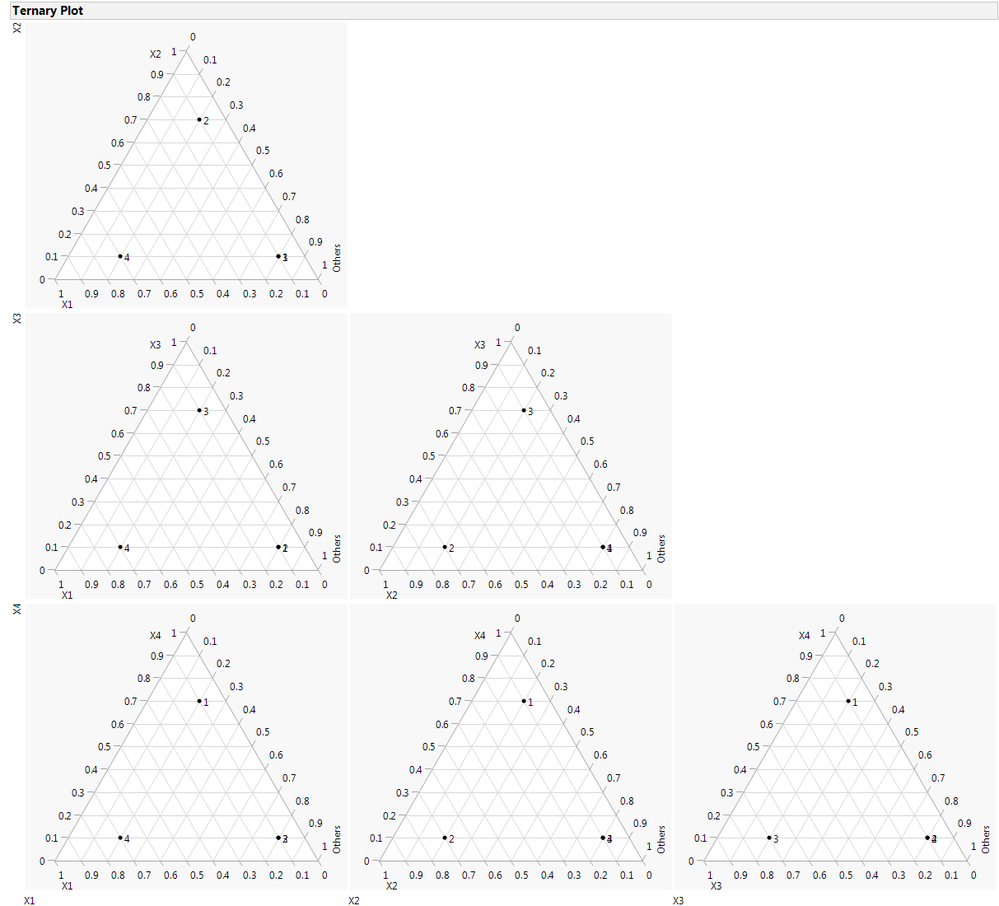Figure 2: Ternary plots of mixtures with three components

### How do we characterize a mixture design space?

In order to build a design space for mixture data, we use an example from Wu et al. (2009). In this experiment, the focus is on the mixing of powders. Key questions for the blending operation include the following:

1. How to quantify components of powder blends simultaneously?
2. How to validate or confirm the process analytic technology (PAT) blending process monitoring results via other fast and convenient spectroscopic methods?
3. How to link the scale of scrutiny and the homogeneity of both API and excipients?

Wu et al. used an extreme vertices design with four components to compute the formulation compositions with the following constraints applied to the weight fractions of corresponding formulation components: for Ibuprofen, 0.25≤wt. fraction≤0.75; for HPMC, 0.01≤wt. fraction≤0.03; for MCC: 0.19≤wt. fraction≤0.57; for Eudragit L 100-55: 0.05≤wt. fraction≤0.15. The JMP Mixture Design platform includes a “linear constraint” option where it is easy to enter the above information. This option can also handle constraints that involve several factors simultaneously, a situation that arises in some mixture designs. The experimental array is presented in Table 1 below with the cells colored by experimental levels (a feature found in JMP column properties).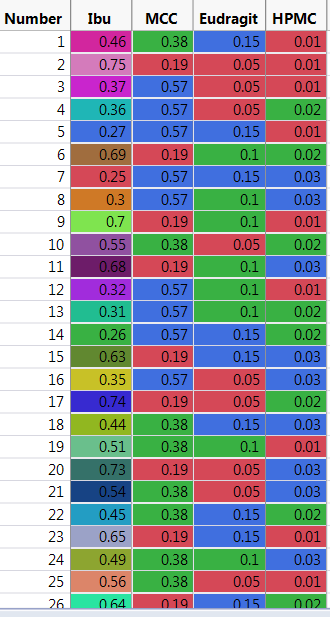Table 1: Extreme vertices design of four components powder mixture experiments

Bulk density: 0.35-0.43 (g/ml)

Tap density: 0.4-0.6 (g/ml)

Mmin: 2.5-3.0 (mg)

Mmax: 13-15 (mg)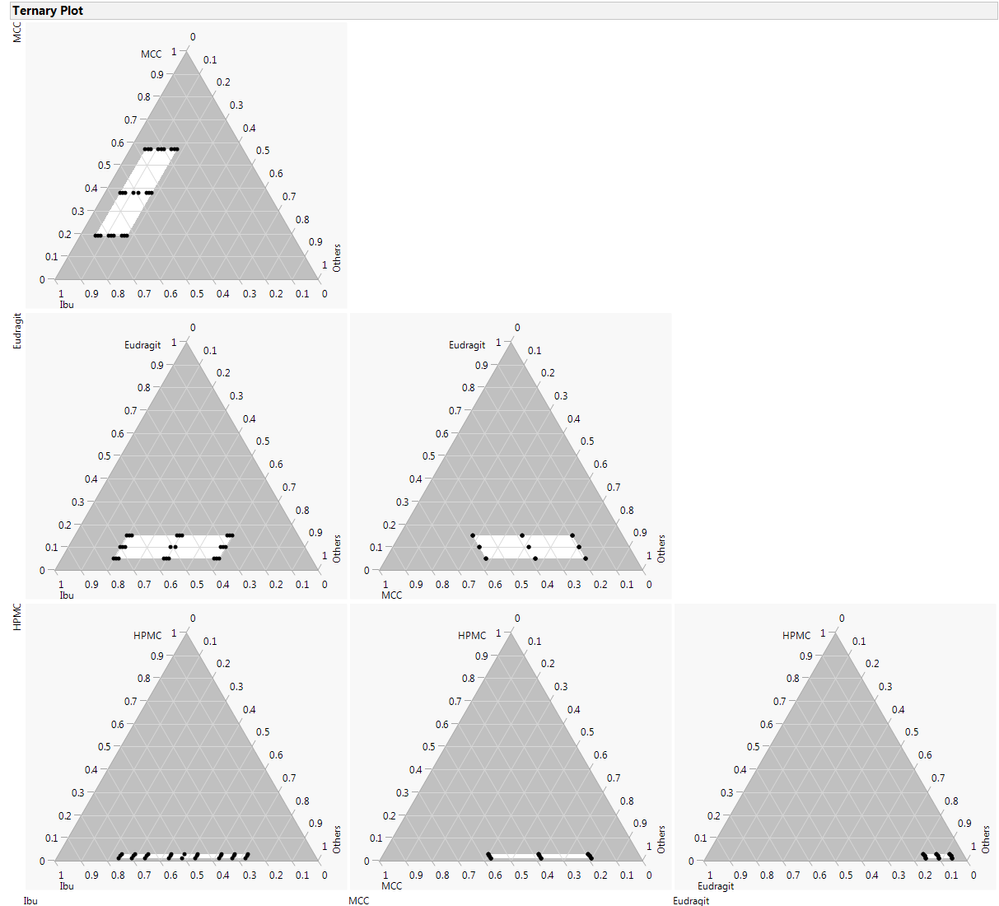Figure 3: Ternary plots of powder mixture experiments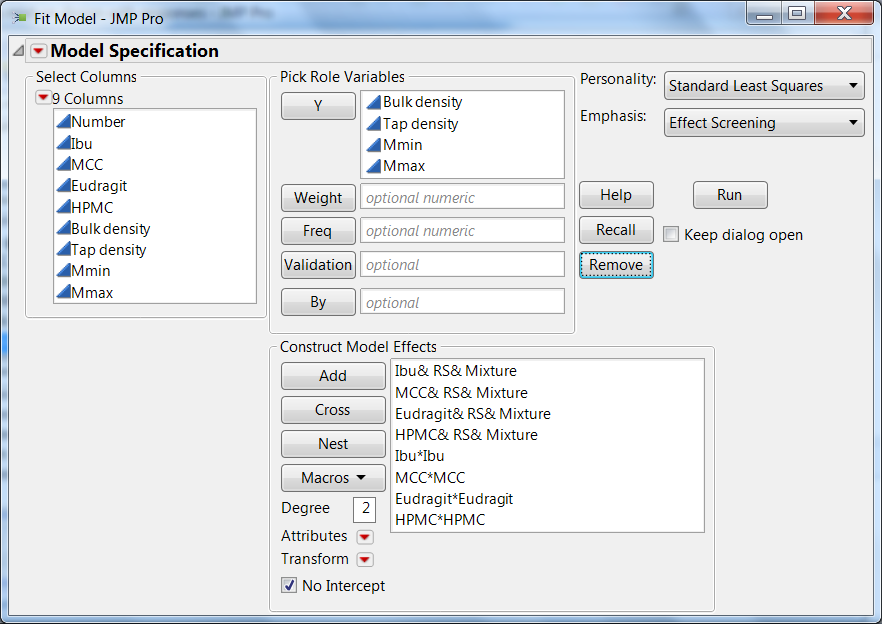Figure 4: Model used in analysis of powder mixture experiments

Figure 5 is a table representing the analysis of Tap density. From the table, we determine that none of the quadratic effects has a significant effect onTap Density. We ran a second analysis, using only the main effects of the four mixture components. Figure 6 shows the results, with statistically significant effects of all four components.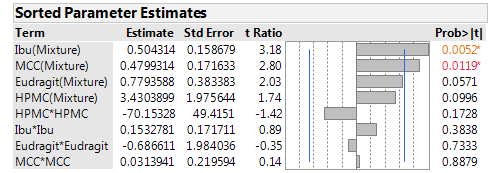Figure 5: Sorted parameter estimates on Tap density of model presented in Figure 4

Figure 6: Sorted parameter estimates on Tap density from the first order model

### Prediction Profiler

The Profiler in JMP provides a quick visual summary of how the components affect all four responses (see Figure 7, from the first order model). The Profiler shows much stronger effects for Ibuprofen and MCC than for Eudragit and HPMC. This is because Ibuprofen and MCC have a much wider range than the other factors.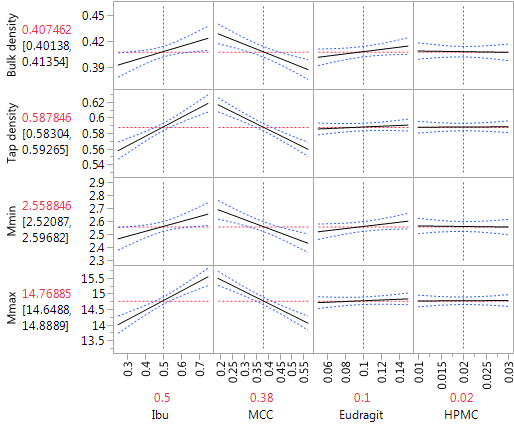Figure 7: Profiler based on first order model used in analysis of powder mixture experiments

We present a contour plot of the responses, based on the ternary representation of Ibu, MCC and Eudragit, in Figure 8.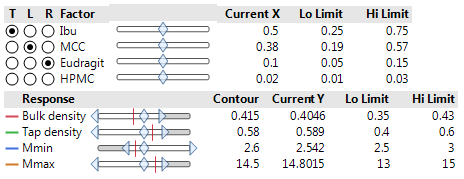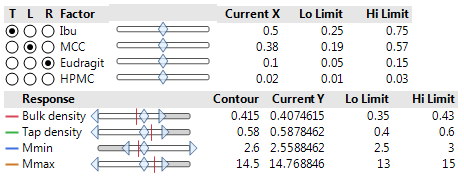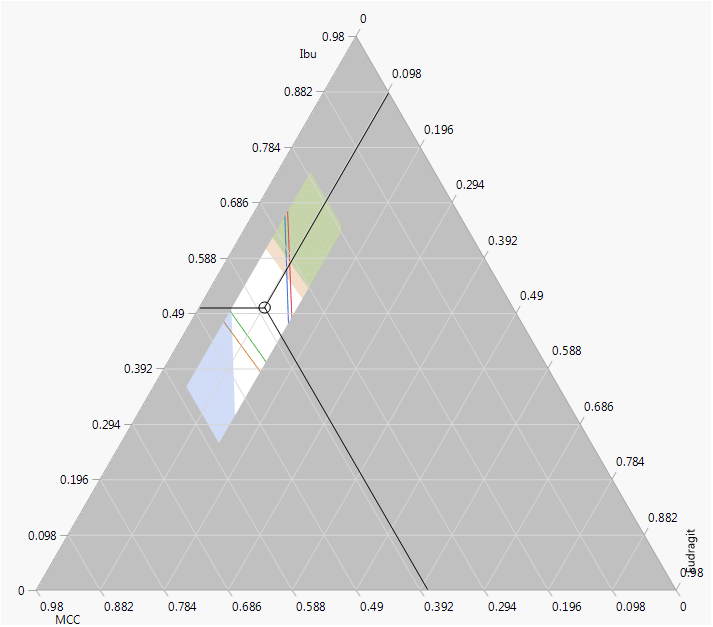Figure 8: Contour plot and design space based on model used in analysis of powder mixture experiments. Current point listed above is marked with a circle

Using the stochastic emulator approach presented in the previous blog post, we derive the Profiler setup presented in Figure 9. In this implementation, the mixture constraints are not enforced so that we need to ensure that simulations are within the design space. In Figure 7, the constraints naturally satisfy this requirement. In other cases, JMP provides an option to perform such simulations.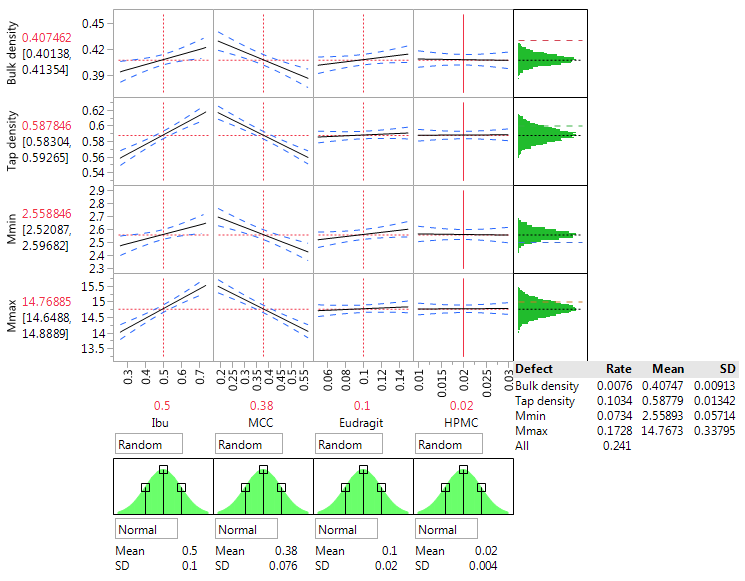Figure 9: Effect of variability of mixture components on model used in analysis of powder mixture experiments

This analysis presents the impact of variability of the components on the responses. With the set up of Ibu=0.5, MCC=0.38, Eudragit=0.1 and HPMC=0.002, and the variability structure described in Figure 8 (means are at set up points and variability with normal distributions and standard deviations determines by experimental range), one gets an overall defect rate of 31%. The Mmax and Mmin responses generated by these simulations have respective means and standard deviations (in brackets) of 14.77 (0.24) and 2.54 (0.04). These two responses induced failure rates of 16% and 14% respectively. In the simulation experiments used in Figure 9, the four factors (components) were sampled independently from their specific variability distributions. JMP also makes it possible to include a correlation structure between the sampled values.

Optimizing on minimal defect rate, as shown in our previous blog post, will lead to a robust formulation around required target values.

### Next in The QbD Column

The next blog post will be dedicated to sequential experimentation strategies by specifically considering a screening design followed by a central composite design.

### Notes

 Wu, H., Tawakkul, M., White, M., Khan, M.. (2009), Quality-by-Design (QbD): An integrated multivariate approach for the component quantification in powder blends, International Journal of Pharmaceutics 372; 39–48.

Article Labels

There are no labels assigned to this post.

Article Tags
1 Comment
Visitor

Manny Uy wrote:

A colleague recently asked me to help him design a mixture experiment. I am not sure how to respond, so I am attaching here his email to me:

I am Nikko Bacalzo in Ateneo de Manila University. I attended your talk here in Ateneo last April 4. I just have a question about cross mixture design.

I am planning to optimize two separate precursor mixtures: A and B, which when mixed, will react to form my desired product. Mixture A can possibly have a combination of three components: A1, A2, A3, at various composition. The same is true for mixture B.

I can make a mixture DOE for each mixture separately using JMP but I can't seem to find how can I possibly cross these two designs. Assuming simplex axial for each mixture, I can have 10 points for each mixture A and B. If I intend to cross the two, I'll have a total of 100 runs (no replicate) for my experiment.

Can JMP process a cross mixture design incorporating 2 separate mixture designs, so that I can minimize (way less than the 'full factorial' 100 runs) the number of runs that I'll have to do?

Thanks a lot,

Nikko"

Manny Uy